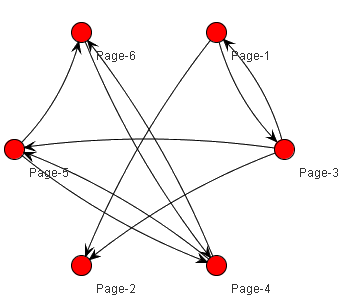### PageRank Implementation Using the BSP

In this post, I'm showing how to implement the PageRank using BSP.
P.S. Apache Hama's BSP framework is not ready yet.

And P.S. again, The pseudo code is developed, based on Java multi-threaded programming. As I introduced before (Hama BSP), the BSP programming is very similar to multi-threaded programming (See BSP serialize printing example). So, the BSP brings a familiar programming model to developers for implementing distributed applications. :-)

Anyway, let's assume that the web-graph G is stored in row sparse format as below:
 Vertex: 1 2 3 4 5 6 Index: 0 1 3 4 6 8 10 IncomingEdgeList: 3 1 3 1 5 6 3 4 4 5 – Vertices V are web pages. – Vertex index[] points to list of incoming edges's vertex.The PageRank Algorithm is as below:

1) If vi links to vk

– User equally likely to follow any link on page.
– Probability of moving from vi to vk = 1/out_degree(vi).


2) If vi has no outlinks…

– User equally likely to jump to any state.
– Probability = 1 / |V|


3) Weighted moves from each page

– Percentage of moves that follow a link == α.
– Percentage of moves that are jumps to another page == (1- α).


Then, the algorithm can be implemented as below:

BSP main program:

BSPPageRank pagerank[] = new BSPPageRank[vertices.length];
// Loop over all vertices
for (i = 0; i < vertices.length; i++) {
pagerank[i] = new BSPPageRank(i);
}

for (i = 0; i < vertices.length; i++) {
pagerank[i].join();
}


Parallel part:

public void run() {
double total = 0.0;
int begin = index[ind];
int end = index[ind + 1];
//System.out.println("incoming edges: " + begin +" ~ "+ end);

// Loop over edges pointing to vertex i.
for (int j = begin; j < end; j++) {
int src = j;
double r = rank[src];
double incr = r / (double) degree[src];
total += incr;
}
accumulate_rank[ind] = total;

// Call sync() method at here, if this is run on the BSP cluster
}


References : MultiThreaded Graph Library (MTGL)

#### 5 comments:

1.I find it a very promising approach!
Can you estimate when Apache Hama's BSP framework will be ready?

2.We have a release plan with BSP (as soon as possible, and at least before the coming autumn).

3.Great news!
Thank you.

4.Hi...

I would be interessted how the follwoing Google Pregel snippet would be implemented using Hama. Simply extending the BSP class as within some of the BSP examples is imo not enough as BSP just models a graph partition and not a single vertex. Is there a more vertex-specifc class anywhere?

// (vertex/edge/message)-type
class MinDistanceVertex
: public Vertex {

// Receive current messages
void Compute(MessageIterator* msgs)
{

// Start of the algorithm
int _MinDist = IsSource(vertex_id()) ? 0 : INF;

// Find smallest value within messages
for (; !msgs->Done(); msgs->Next())
_MinDist = min(_MinDist, msgs->Value());

if (_MinDist < GetValue())
{

// Set internal value to new minimal distance
*MutableValue() = _MinDist;

// Send new minimal distance to neighboring nodes
SendMessageToAllNeighbors(_MinDist + 1);

}

// Superstep Synchronization
VoteToHalt();

}

}

5.The exhibited code does not handle the case of a sink

### 무한의 세계

무한 집합의 크기 Cardinality , 즉 원소의 개수를 수학에서는 '농도'라고 말한다. 유한 집합의 크기는 그대로 원소의 개수 이지만, 무한 집합의 경우는 원소의 개수를 낱낱이 셈하는 것은 불가능하기 때문에 '농도'라...